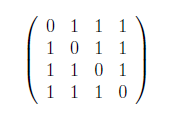Related Articles
GATE | GATE CS 2021 | Set 1 | Question 62
• Last Updated : 06 Apr, 2021

Consider the following matrix.The largest eigenvalue of the above matrix is __________.
(A) 3
(B) 4
(C) 0
(D) 12

Answer: (A)

Explanation: Either you follow brute force approach Or

Shortcut-: When each row sum is equal that would be the maximum Eigen value of the matrix. In this case sum is 3 so answer will be 3.

Quiz of this QuestionMy Personal Notes arrow_drop_up